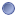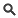### Module Detail Information

 Name: ShapeDistanceType:Module Short URL: `http://bit.ly/1DjhgWQ` Description: A program to calculate the distance between homologous vertices in a pair of Shapes. This program computes the distance between the vertices of two Shapes. The homology between vertices is based upon the order in which the vertices are added to the Shape's point sets; If you compare shapes A and B, the first distance value is that between vertex A_0 and vertex B_0. Thus, the Nth distance value is that between vertices A_n and B_n. The distances so computed are written to an output file. Two output formats are supported (version 1.0.0a0) : a simple text file, with one distance per line, and a LONI Ucf file, in which the distances are appended as a data attribute to the first shape given as an argument to the ShapeDistance application. Two distance metrics are currently supported: Euclidean and Taxicab. The second shape file may contain more vertices than the first file. If this is true the extra vertices are ignored, unless the -strict flag is enabled, in which case the number of vertices in the two files must be equal. Note The current default output type is a text file. The file type is not automatically derived from the input files. Executable: `/java` Input Parameters: - Jar  - -format  - -distance  - -strict  - -attribute  - -files  - -help  - -version  - -verbose  - -license  - -twiki Output Parameters: - -output File size: 29.71 KBView SourceDownloadOpen